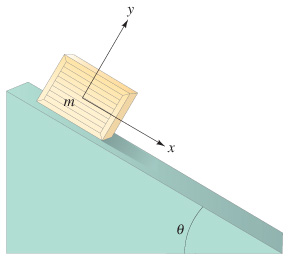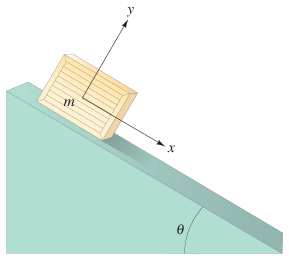# Problem: A crate is given an initial speed of 3.0 m/s up the 28° plane shown in the figure. Assume μk = 0.16.(a) How far up the plane will it go?(b) How much time elapses before it returns to its starting point?

###### FREE Expert Solution

We're asked for the maximum distance the crate gets from its starting point and the time it takes to return to the starting point.

For inclined-plane problems that involve friction, here's what we'll do:

1. Draw a free body diagram (FBD) using tilted coordinate axes
2. Apply Newton's 2nd Law ( Fma )
3. Apply kinematics (if needed)
4. Solve for the target variable

Problems like this can look complicated at first, but once you break them down, they're just like other problems we've worked on before!

The kinetic friction force is calculated using

$\overline{){{\mathbit{f}}}_{{\mathbit{k}}}{\mathbf{=}}{{\mathbit{\mu }}}_{{\mathbit{k}}}{\mathbit{N}}}$

The four kinematics equations are

Also remember that when we use tilted coordinate axes for inclined plane problems like this, the weight mg makes a known angle with the y-axis, not the x-axis. That means we decompose the weight vector like this:

95% (283 ratings)###### Problem Details

A crate is given an initial speed of 3.0 m/s up the 28° plane shown in the figure. Assume μk = 0.16.

(a) How far up the plane will it go?

(b) How much time elapses before it returns to its starting point?